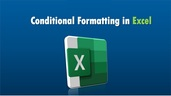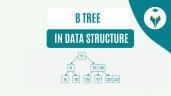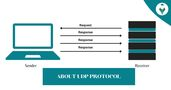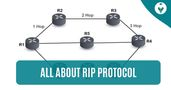Relative Referencing in Excel – Shiksha Online

# Relative Referencing in Excel – Shiksha Online

clickHere
Updated on Mar 3, 2023 13:34 IST

The article covers the Relative Referencing in Excel, how to create a relative reference, and examples of relative referencing.

Relative references refer to a range of cells or an individual cell in Excel. Every time we input a value into a formula, such as COUNTIF or SUMIF, it is possible to input a “cell reference” into Excel as a substitute for a hard-coded number. A cell reference may come in A2, where A refers to the column, and 2 refers to the row number. To understand this further, read this blog on Relative Referencing in Excel.

Content

## How to Create a Relative Reference in Excel

Whenever Excel deals with a cell reference, it visits the cell in question. It further extracts the cell value and uses that specific value in the formula created. If you duplicate this cell reference to another location, the cell’s relative references also automatically change.

When we reference cells in this way, we get two types of references, relative and absolute. The difference between these two references is that they demonstrate different inherent behavior when dragging, copying, and pasting in nearby cells.

Relative references can alter and adjust when copy-pasted, while absolute references do not. To successfully achieve results in Excel, you must use relative and absolute references accurately.

Purpose – Relative reference gives flexibility to the formulas. If you change the value of one of the cells, the value that the reference takes will automatically be adjusted. In addition, being automatic, errors are avoided, and time is saved.

We can use relative references in the following way, for example, by making a formula containing the relative reference A1 multiplied by a value. In this case, we will multiply it by three, and it would be as follows =A1*3

Where A1 is our relative reference containing a value and will be multiplied by 3, our value is chosen as an example.

The benefit of relative references in Excel is that they get automatically modified when copying them to another cell.

The cell where you have introduced reflective reference will change as you copy the formula to another cell. What Excel understands is the relative position of the cells. It is easier to understand with the following examples.

## Example 1 – Relative Referencing

We have a range in which we have the hourly pay and the hours worked to obtain the total payment for each worker.

So, we need to multiply the hourly rate by the hours worked. For that, we write =B2*C2 in cell D2

To fill the rest of the data range, we must drag the formula using the lower right corner of cell D2 or by double-clicking on this same corner.

As per the illustration, Jonas worked 3 hours and was paid \$13 per hour. So his total payment is \$39. We see that the formula in D4 is =B4*C4. This is a relative reference: Excel remembers the formula as “multiply the cell to the left by the cell two to the left.”Most Popular and Powerful Formulas in Excel
The article discusses the most popular formulas in excel through practical examples of the use of formulas for Excel.Average Function in Excel – Formula and Examples
The AVERAGE function is a popular Excel function used for various purposes, such as calculating average sales, average student scores, average monthly expenses, etc. It provides a quick and efficient...read moreWhat is Conditional Formatting in Excel?
Learn what is Conditional Formatting in Excel and explore different presets of Conditional Formatting to analyze and present your data in the most effective and powerful way.

## Example 2 – Relative Referencing

Consider another example. We use the SUM formula that adds the total cost or value of the products in January in cell B7.

Cell B7 is a relative reference that contains a formula summing up the values ​​of B2:B6.

We will copy or replicate the formula in other fields to get the total. When dragging B6 through F6, we will notice that we will be increasing the columns. This way, the references of these cells will change according to their corresponding ranges.

When copying the formulas to other cells, Excel will automatically adjust the references; that is, it will modify the columns and rows corresponding to each formula range. In addition, this will be relative to the number of columns we have moved.Top MS Excel Interview Questions
Master your Excel interview with confidence using our curated list of top questions. From basic functions to advanced data analysis, boost your Excel proficiency and shine in any data-driven role.Introduction to MS Excel – A Beginner’s Guide
Let’s gets started with MS Excel, the most useful tool to crunch numbers and derive information for data analysts and scientists.How to Create MIS Reports in Excel?
MIS reports are crucial to measure the business performance and contribute towards meeting the overall business goals. In this article, you will learn easy steps to create an MIS report...read more

## Points to Remember

• In relative referencing, the referred cells automatically set themselves in the formula when moved across any direction.
• If we give reference to B10 and shift to the next cell, it would change to B11. If we move one cell upward, the relative reference will be B9. When we move to the right, the reference will be C10, and so on.

I hope this article helped you understand Relative Referencing in Excel and how it works. In the following article, we will cover the concept of absolute referencing in Excel

## FAQs

What are absolute and relative references in Excel?

There are two types of references: relative and absolute. Relative references change when they are copied from one cell to another. Absolute references remain constant no matter what cell they are copied to.

What are relative references in Excel?

Relative references refer to the location of the cell. Relative references allow Excel to modify the column and row when copying the formula to other cells. The change will be relative to the number of columns or rows the formula has shifted.

How do I turn on relative references in Excel?

When you write a formula in Microsoft Excel, press the F4 key. This allows switching between relative, absolute, and mixed cell references.

What are absolute and mixed relative references?

Absolute references will be applied when neither the column nor the row is going to change; relative references when only one column or row is going to change, and mixed references when combining relative with absolute.

How to make an absolute reference in Excel without F4?

To work with absolute references, it must be specified by writing the \$ sign in front of the column letter and the row number.

clickHere

Rashmi is a postgraduate in Biotechnology with a flair for research-oriented work and has an experience of over 13 years in content creation and social media handling. She has a diversified writing portfolio and aim... Read Full Bio

## Trending Management CoursesExcel for Beginners
Great Learning4.6Microsoft Excel - Excel from Beginner to Advanced
UDEMY4.7Introduction to MS Excel
Simplilearn4.6Create Charts and Dashboards Using Microsoft Excel
Coursera4.0Starts todayUsing Basic Formulas and Functions in Microsoft ExcelUsing Basic Formulas and Functions in Microsoft Ex...
Coursera5.0Starts today

## Top Picks & New Arrivals